Geometry 6-8 Applying Coordinate Geometry
starstarstarstarstarstarstarstarstarstar
by Matthew Richardson
| 21 Questions
Note from the author:
A complete formative lesson with embedded slideshow, mini lecture screencasts, checks for understanding, practice items, mixed review, and reflection. I create these assignments to supplement each lesson of Pearson's Common Core Edition Algebra 1, Algebra 2, and Geometry courses. See also mathquest.net and twitter.com/mathquestEDU.
The outlined content above was added from outside of Formative.
The outlined content above was added from outside of Formative.
1
10 pts
Solve It! The points shown are three vertices of a parallelogram. Draw all the possible locations of D, the fourth vertex?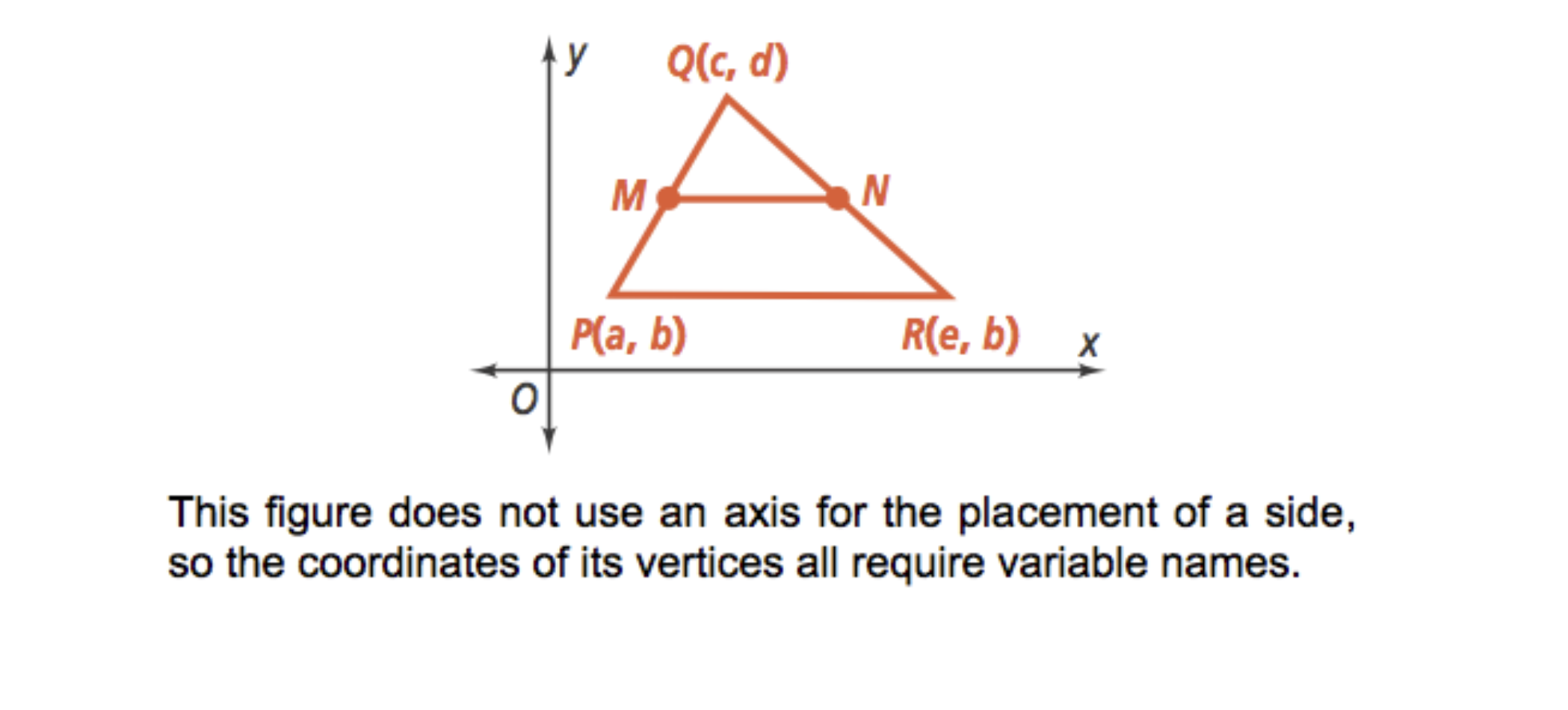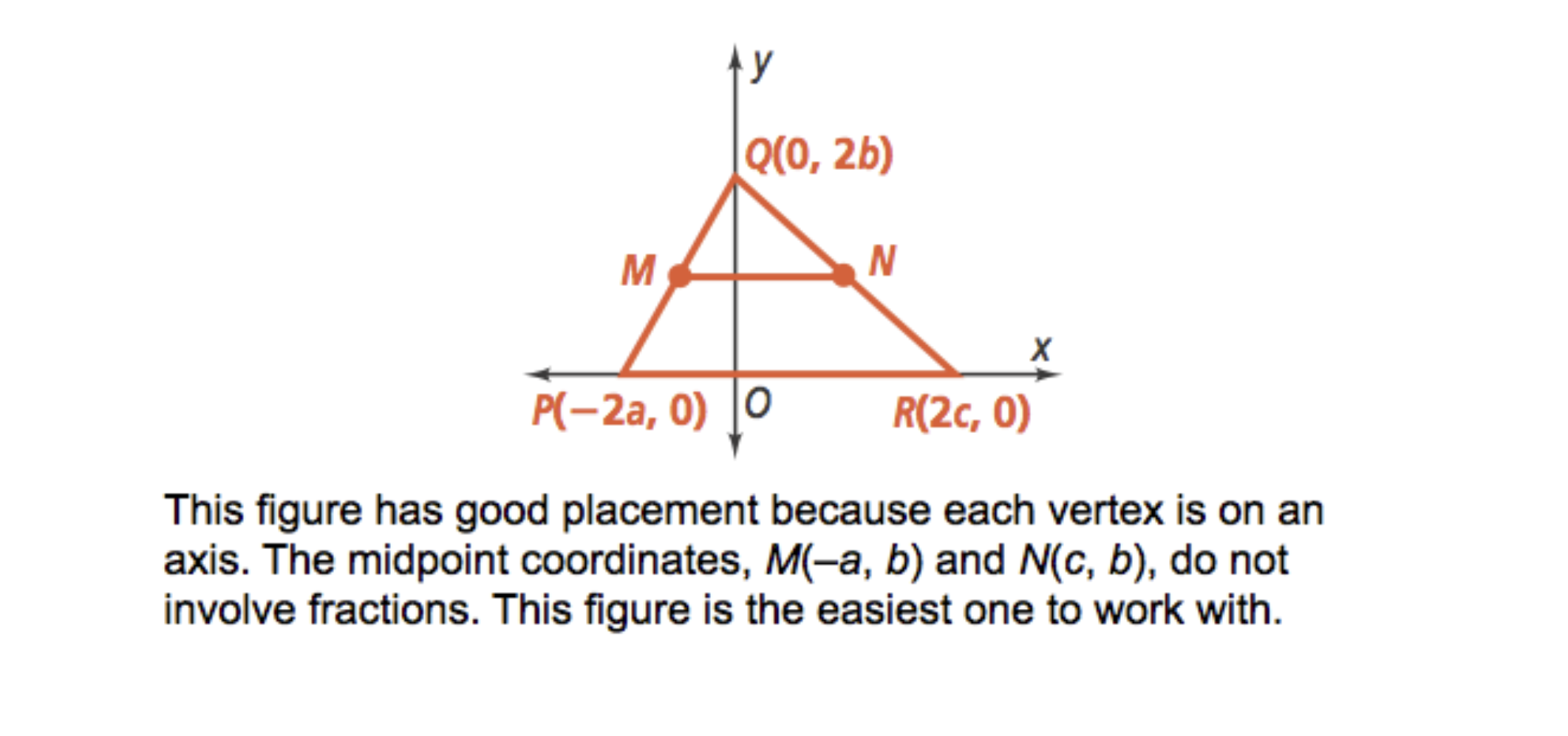The outlined content above was added from outside of Formative.2
2
10 pts
Problem 1 Got It?
A
B
C
D3
3
10 pts
Problem 1 Got It?
A
B
C
D
The outlined content above was added from outside of Formative.45
5
10 pts
Problem 2 Got It. The diagram shows a trapezoid with the base centered at the origin. Is the trapezoid isosceles? Explain.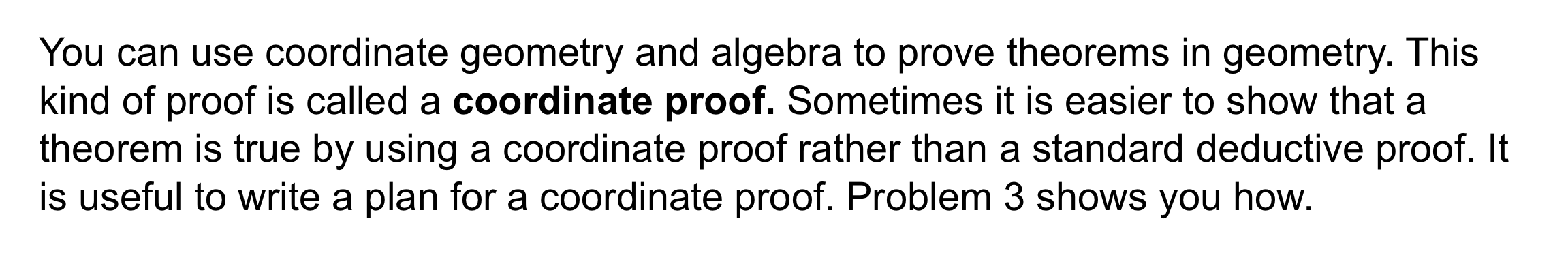The outlined content above was added from outside of Formative.6
6
10 pts
Problem 3 Got It? Plan a coordinate proof of the Triangle Midsegment Theorem (Theorem 5-1).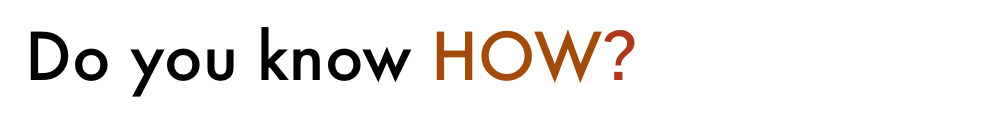7
7
10 pts
A
B
C
D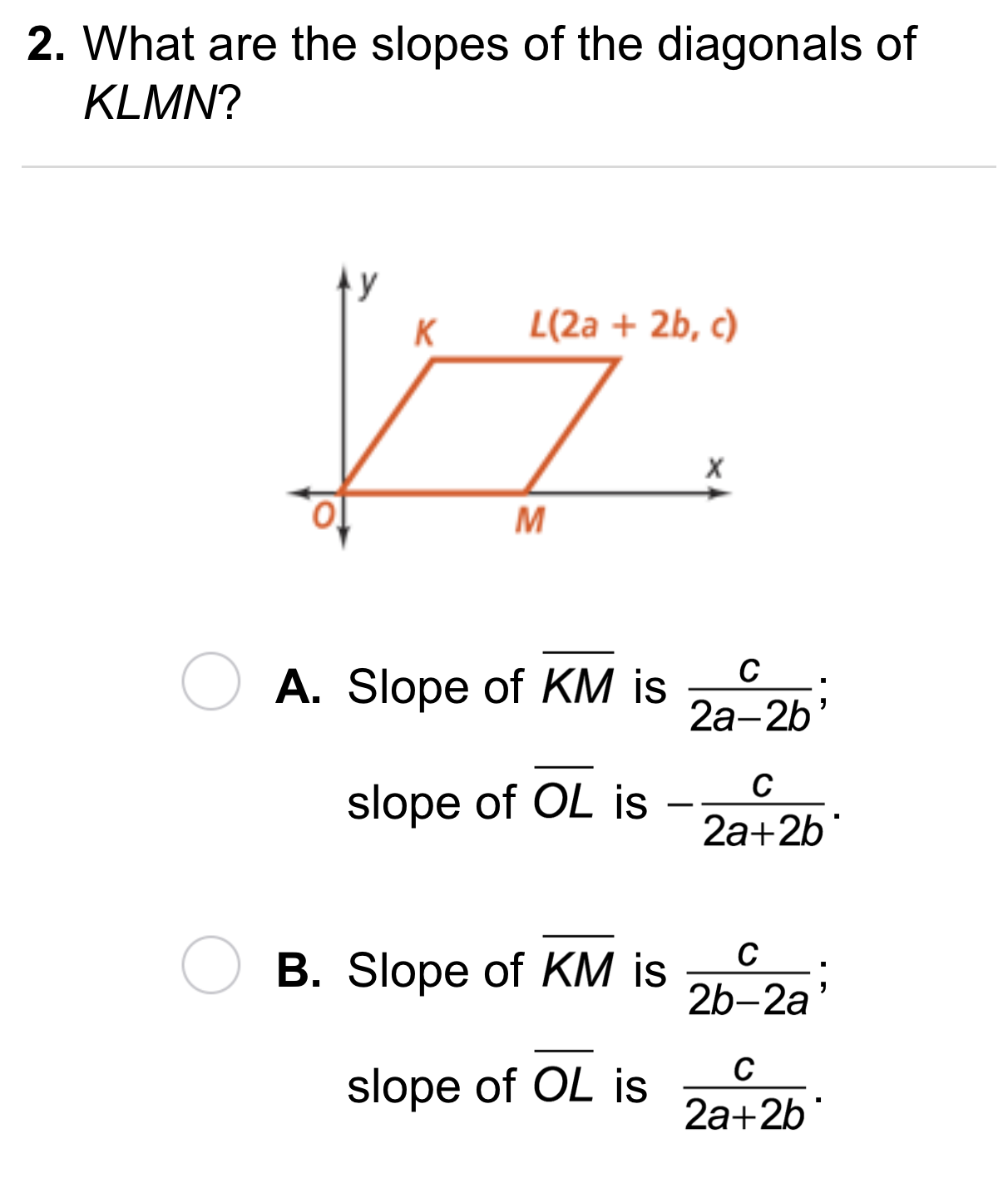8
8
10 pts
A
B9
9
10 pts
A
B
C
D10
10 pts
Reasoning: How do variable coordinates generalize figures in the coordinate plane? For example, what do you know about (3, a), (b, -5), etc.
11
10 pts
Reasoning: A vertex of a quadrilateral has coordinates (a, b). The x-coordinates of the other three vertices are a or -a and the y-coordinates are b or -b. What kind of quadrilateral is the figure? Be as specific as possible.
The quadrilateral is a rectangle.
The quadrilateral is a rhombus.
The quadrilateral is a square.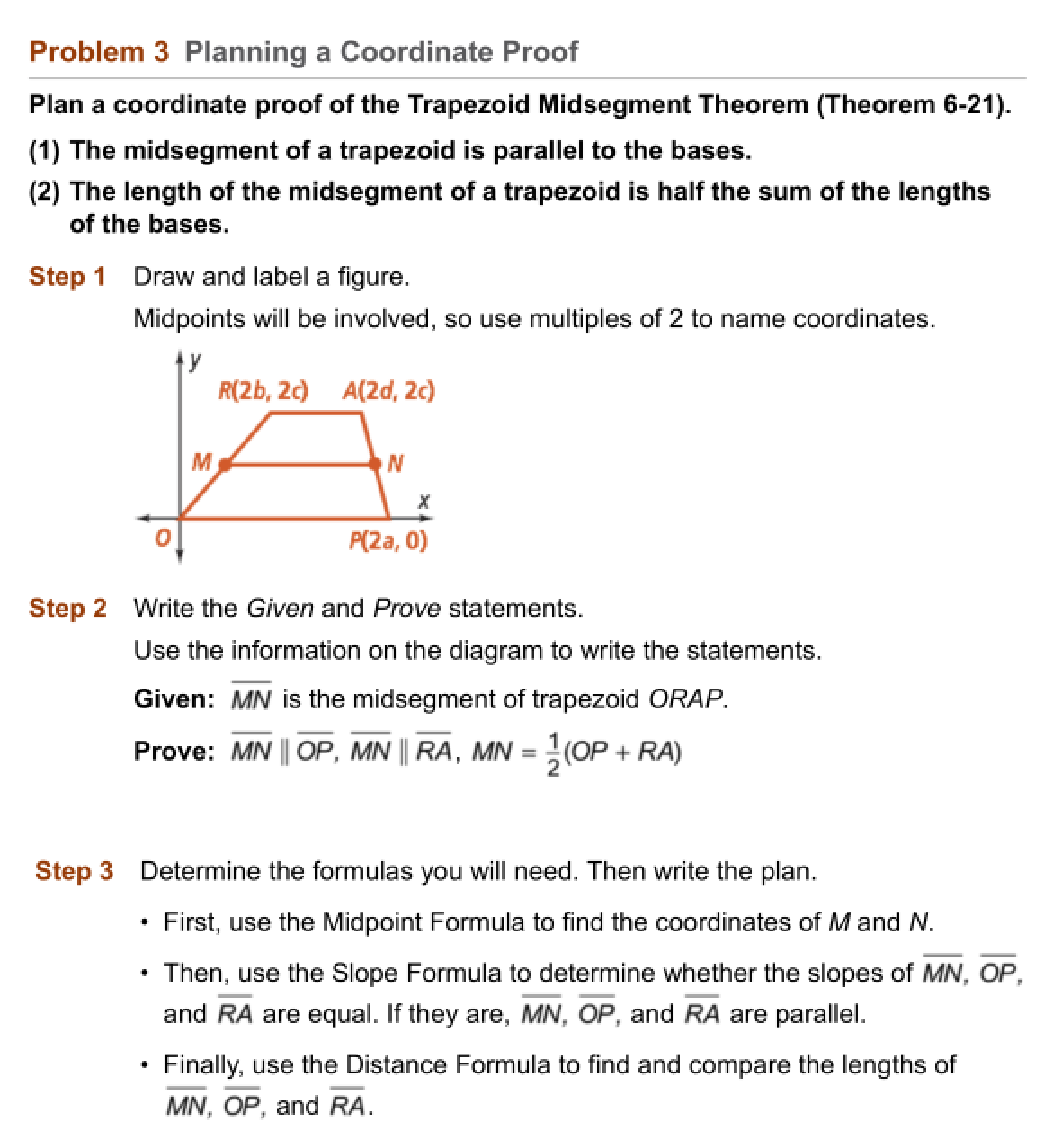12
12
10 pts
Error Analysis: A classmate says the endpoints of the midsegment of the trapezoid in Problem 3 are as follows.
What is your classmate's error? Explain.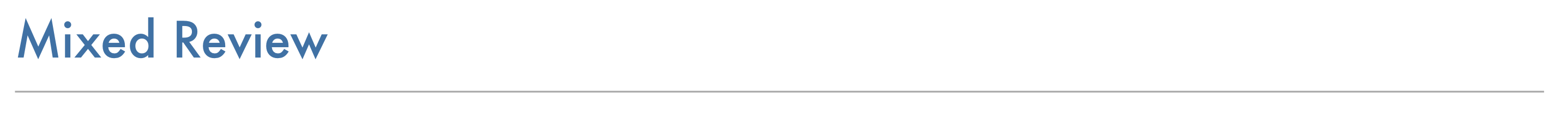13
10 pts
Review Lesson 6-7: Let X(-2, 3), Y(5, 5), and Z(4, 10) be the vertices of a triangle. Is △XYZ a right triangle? Explain.
14
10 pts
Review Lesson 2-2: Write the inverse of the statement.

If x = 51, then 2x = 102.
15
10 pts
Review Lesson 2-2: Write the converse of the statement.

If x = 51, then 2x = 102.
16
10 pts
Review Lesson 2-2: Write the contrapositive of the statement.

If x = 51, then 2x = 102.
17
10 pts
Review Lesson 3-7: Find and graph the equation of the line that contains the origin and (4, 5).
18
10 pts
Review Lesson 3-7: Find the equation of the line that contains (p, q) and has slope a/b.
y - p = (a/b)(x - q)
y - q = (a/b)(x - p)
y - a = (p/q)(x - b)
y - b = (p/q)(x - a)19
10 pts
Vocabulary Review: Categorize each statement as true or false.
• True
• False
• The vertex of an angle is the endpoint of two rays.
• When you name angles using three points, the vertex gets named first.
• A polygon has the same number of sides and vertices.20
20
10 pts
Use Your Vocabulary: Match each point with its coordinates.
• A
• B
• C
• D
• (-1, -3)
• (1, 3)
• (3, -1)
• (-3, 1)
21
10 pts
Reflection: Math Success
Add to my formatives list

Formative uses cookies to allow us to better understand how the site is used. By continuing to use this site, you consent to the Terms of Service and Privacy Policy.